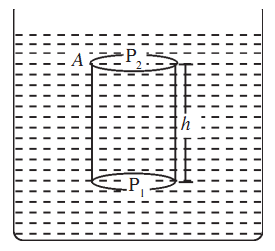# Hydrostatic Pressure

For the same force, the effect is greater for smaller area. This effect of force on unit area is called pressure. The normal force or thrust per unit area exerted by a fluid is called pressure.

P = Thrust / area

The pressure exerted by a fluid at rest is known as hydrostatic pressure. The SI Unit of pressure is Nm–2 and is also called pascal (Pa).

### Hydrostatic Pressure at Point Inside Liquid

Consider a liquid in a container and an imaginary right circular cylinder of cross sectional area A and height h. Let the pressure exerted by the liquid on the bottom and top faces of the cylinder be P1 and P2, respectively.The upward force exerted by the liquid on the bottom of the cylinder is P1A and the downward force on the top of the cylinder is P2A.

∴ The net force in upward direction is (P1A – P2A).

Mass of the liquid in cylinder = density × volume of the cylinder

= ρ. A. h

where ρ is the density of the liquid.

∴ Weight of the liquid in the cylinder = ρ. g. h. A

Since the cylinder is in equilibrium, the resultant force acting on it must be equal to zero.

P1A – P2A – ρ g h A = 0

P1 – P2 = ρ g h

So, the pressure P at the bottom of a column of liquid of height h is given by

P = ρ g h

Hydrostatic pressure due to a fluid increases linearly with depth. It is for this reason that the thickness of the wall of a dam has to be increased with increase in the depth of the dam.

If you consider the upper face of the cylinder to be at the open surface of the liquid, then P2 will have to be replaced by Patm (atmospheric pressure). If you denote P1 by P, the absolute pressure at a depth below the surface will be

P – Patm + ρ g h = 0

P = Patm + ρ g h

Pressure in a liquid at a given depth is equal, irrespective of the shape of the vessel.